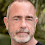## Monday, May 9, 2016

### Cover Reveal: A Dangerous Game by Rick R. Reed #Excerpt #Giveaway=•=•=•=•=•=•=•=•=•=•=•=•=•=•=•=•=•=•=•=•=•=•=•=•=•=•=•=•=

Rick R. Reed reveals the beautiful cover art of his next book titled A DANGEROUS GAME coming out from Dreamspinner Press.

It releases on June 03, 2016.

=•=•=•=•=•=•=•=•=•=•=•=•=•=•=•=•=•=•=•=•=•=•=

BLURB

2nd Edition

Sex can be a dangerous business. So can love.

On the worst day of his life, Wren Gallagher wants oblivion when he steps into Tricks for a drink. When a mysterious stranger steps up to pay his tab, he offers Wren the key to fulfilling his dreams of prosperity and true love.

But appearances are not always what they seem.

His savior owns the escort agency À Louer, and he wants the young and handsome Wren as part of his stable of men-for-hire. Down on his luck, Wren figures, why not? He needs the money. When he joins, though, he doesn’t count on meeting Rufus, another escort with whom he falls hopelessly in love.

But their love story will have to overcome the obstacles of not only trading love for money, but À Louer’s dark—and deadly—secrets.

1st Edition published as Rent by ManLove Romance Press, 2012.

=•=•=•=•=•=•=•=•=•=•=•=•=•=•=•=•=•=•=•=•=•=•=•=•=•=•=•=•=

PRESENTING

Cover Art by Reese Dante=•=•=•=•=•=•=•=•=•=•=•=•=•=•=•=•=•=•=•=•=•=•=

EXCLUSIVE EXCERPT

“How’d you get so cute?”
Rufus finally broke the silence. Wren could have easily fired back the same question. Rufus was what his mother would have called a “doll.” He stood a few inches taller than Wren, maybe six one or six two, and his head was topped with a thatch of wheat-colored hair that fell fetchingly across his forehead, every so often blocking the view of one of his startlingly dark blue eyes. The color reminded Wren of sapphires. He had pale skin, a lanky frame—that promised, Wren knew from past experience, a big dick—and a dusting of pale hair on his chin and upper lip that served to make him only look more masculine and sexy.

Read more exclusive excerpts on –

=•=•=•=•=•=•=•=•=•=•=•=•=•=•=•=•=•=•=•=•=•=•=

=•=•=•=•=•=•=•=•=•=•=•=•=•=•=•=•=•=•=•=•=•=•=Rick R. Reed is all about exploring the romantic entanglements of gay men in contemporary, realistic settings. While his stories often contain elements of suspense, mystery and the paranormal, his focus ultimately returns to the power of love.

He is the author of dozens of published novels, novellas, and short stories. He is a three-time EPIC eBook Award winner (for Caregiver, Orientation and The Blue Moon Cafe). He is also a Rainbow Award Winner for both Caregiver and Raining Men. Lambda Literary Review has called him, "a writer that doesn't disappoint."

Rick lives in Seattle with his husband and a very spoiled Boston terrier. He is forever "at work on another novel."

=•=•=•=•=•=•=•=•=•=•=•=•=•=•=•=•=•=•=•=•=•=•=

RAFFLECOPTER GIVEAWAY

Winner’s Prize: Winner’s choice of any eBook title from Rick’s Dreamspinner Press backlist.

1.Thank you for showcasing Reese Dante's gorgeous cover. I just wanted to let folks know that this week, if you pre-order A DANGEROUS GAME, you can get it at 35% off!

2.I'd rather win this title but there are a few of Rick's backlist which I am still to get.

3.Very beautiful cover! Looking forward to reading it!

juliesmall2016(at)gmail(dot)com